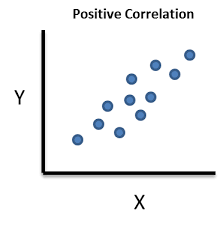# Correlational Designs

Correlational research is focused on examining the relationships among two or more variables. This information can be used either to explain a phenomenon or to make predictions. This post will explain the two forms of correlational design as well as the characteristics of correlational design in general.

Explanatory Design

An explanatory design seeks to determine to what extent two or more variablesco-vary. Co-vary simply means the strength of the relationship of one variable to another. In general, two or more variables can have a strong, weak, or no relationship. This is determined by the product moment correlation coefficient, which is usually referred to as r. The r is measured on a scale of -1 to 1. The higher the absolute value the stronger the relationship.

For example, let’s say we do a study to determine the strength of the relationship between exercise and health. Exercise is the explanatory variable and health is the response variable. This means that we are hoping the exercise will explain health or you can say we are hoping that health responds to exercise. In this example, let’s say that there is a strong relationship between exercise and health with an r of 0.7. This literally means that when exercise goes up one unit, that health improves by 0.7 units or that the more exercise a person gets the healthier they are. In other words, when one increases the other increase as well.

Exercise is able to explain a certain amount of the variance (amount of change) in health. This is done by squaring the r to get the r-squared. The higher the r-squared to more appropriate the model is in explaining the relationship between the explanatory and response variable. This is where regression comes from.

This also holds true for a negative relationship but in negative relationships when the explanatory variables increase the response variable decreases. For example, let’s say we do a study that examines the relationship between exercise and age and we calculate an r of -0.85. This means that when exercise increases one unit age decreases 0.85. In other words, more exercises mean that the person is probably younger. In this example, the relationship is strong but indicates that the variables move in opposite directions.

Prediction Design

Prediction design has most of the same functions as explanatory design with a few minor changes. In prediction design, we normally do not use the term explanatory and response variable. Rather we have predictor and outcome variable as terms. This is because we are trying to predict and not explain. In research, there are many terms for independent and dependent variable and this is because different designs often use different terms.

Another difference is the prediction designs are focused on determining future results or forecasting. For example, if we are using exercise to predict age we can develop an equation that allows us to determine a person’s age based on how much they exercise or vice versa. Off course, no model is 100% accurate but a good model can be useful even if it is wrong at times.

What both designs have in common is the use of r and r square and the analysis of the strength of the relationship among the variables.

Conclusion

In research, explanatory and prediction correlational designs have a place in understanding data. Which to use depends on the goals of the research and or the research questions. Both designs have